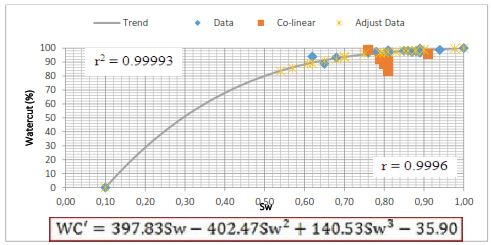# Analyzing The Statistics Function For Determination Of Oil Flow Rate Equation in New Productive Zone

• Ira Herawati Petroleum Department, Faculty of Engineering, Islamic University of Riau, Riau, 28284,Indonesia
• Novia Rita Petroleum Department, Faculty of Engineering, Islamic University of Riau, Riau, 28284,Indonesia
• Novrianti Novrianti Petroleum Department, Faculty of Engineering, Islamic University of Riau, Riau, 28284,Indonesia
• Rosalia M Taufand Petroleum Department, Faculty of Engineering, Islamic University of Riau, Riau, 28284,Indonesia
Keywords: Oil Rate, Statistical Analysis, Coefficient Determination, Productivity Index, Watercut

### Abstract

Oil rate will be decline at production time in a well. So, we have to produce in another layer who assume have a potential. Before we produce another layer who assumed have a potential, we need to predict oil rate to known how much oil gain. In this field research oil rate prediction in new productive zone was determine following by analogical data and near well references. In this method there is a difference determine of oil rate for each people. Cause of that, in this research using analysis statistical for oil rate predicting in new productive zone based on linear function for Productivity Index (PI) and polynomial function for watercut. Determining equation of linear and polynomial functions for oil rate prediction measuring by production and logging data for each well who assumed productive zone in area X field RMT. Based of statistically analysis for linear function known that coefficient determination (r2) = 0.9964 and polynomial function known that coefficient determination (r2) = 0.9993. This result indicated that we can use both of the functions for oil rate prediction in new productive zone in area X field RMT. After that, based on both of functions calculate oil rate prediction each wells in area X field RMT. So, known differences in oil rate prediction between oil rate data in area X field Y known is 28.13 BOPD or 0.78%.

### References

Ariadji, Tutuka. Radjes, Muhammad Tities. 2012. “Kuantifikasi Ketidakpastian Pengembangan Lapangan Secara Terintegrasi Surface Dan Subsurface Dengan Menggunakan Desain

Ariadji, Tutuka. Radjes, M.T., 2012. Kuantifikasi Ketidakpastian Pengembangan Lapangan Secara Terintegrasi Surface dan Subsurface dengan Menggunakan Desain Eksperimenta. IATMI.
Jothikumat, E. al., 2004. AP ® Statistics.
Kelkar, 2002. Applied Geostatistics for Reservoir Characterization.pdf.

Morrison, F.A., 2015. Obtaining Uncertainty Measures on Parameters of a Polynomial Least Squares Fit with Excel ’ s LINEST 1–7.

Stroud K.A and J. Dexter, 2003. advanced-engineering-mathematics-4th.

Schmuller, Joseph. 2013. Statistical Analysis with Excel Third Edition. Hoboken, New jersey

Stanley, L.T. 1973. Practical Statistic for Petroleum engineers. Petroleum Publishing Company. Tulsa

Tang, hong. 2007. Using production Data To mitigate reservoir Connectivity uncertainty. Lousiana State university. International Petroleum Technology.Published
2017-03-01
Section
Research Articles
Abstract viewed = 133 times